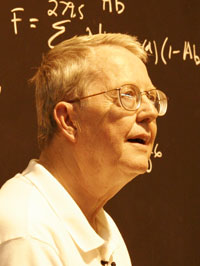### The Graviton ExperimentThe Graviton Experiment
Robert de Hilster

Abstract
This paper describes and experiment which attempts to prove that the graviton or gravity quantum exists by using the concepts introduced by Argentinean physicist Dr. Ricardo Carezani. The experiment tries to find a difference between Newton’s empirical
gravity equation and the equation developed for the finite quanta the graviton. The results of the predictions by the graviton equations give different results depending on the experimental setup. Several differences in the equations are discussed along with a general discussion of possible experimental setups. Although one experiment was performed, the setup had inherent difficulties and could not yield any conclusions. Variations of this experiment are proposed, but there may be other experiments that are easier to do.

### Eine Antwort zu “The Graviton Experiment”

1. Helmut Hille

Gravity and Quantum Physics
Note from June 2011

Here a summary of what we definitely know about the gravity and soundly can say:

1. The gravity exists.
2. The gravity cannot be shielded, what is already an important indication of its nature.
3. Belonging to the particle/body, it is spreading itself evenly as gravitational field round the space.
4. Any object has gravity and is subjected to it.
5. While falling in a gravitational field not the nature of the falling object but its local force and resulting from it direction is what counts.
6. The ratio of the mass/energy of an object and its gravitational force is constant (gravitational constant).
7. What we as well might know in the quantum physics age: also the gravitational force is an entanglement phenomenon caused by so called Big-Bang of the inhabited by us universe and because of it in its force determined.
8. The entanglement phenomenon has no time factor.
9. Thus the gravity is from the beginning at the place of its work, it must neither rush first through the space nor ‚bend‘ itself beforehand.
10. I think that the gravity range of an object is restricted by the Planck constant. That means that field cannot reduce itself as much as it would like to. This fact contributes to the increasingly accelerated expansion of the cosmos, as evidenced by ever-faster ‚escape‘ of its quasars and galaxies, which is already unavoidable due to reduction of the gravity in the intergalactic space.

Therefore there is not only no problem between the gravity and the particles, on contrary: only quantum physics makes us understand the gravity and the cosmos. And that is in my opinion all what we, within the meaning of the quantum physics positivism based on the observable, definitely know and can soundly say and it is also all we need to know and what should be understood. All other assumptions of the ‚explanation‘ of the gravity which are going beyond it are in my opinion pure and unnecessary fiction.
In this context I would lie to recall what Carl Friedrich von Weizsäcker* hoped on the 49th Physicists Conference in Munich in 1985 on the occasion of the Niels Bohr’s centenary birthday: „But in exact reverse of Einstein’s hope I would assume that this (in future comprehensive) theory would not profit from the reduction of the quantum theory of the space-time-continuum, but from derivation of the space-time-continuum as classic borderline case of the pure quantum theory.“ By this the sadly missed unity of physics will be re-established and will make Einstein’s assumptions redundant.
*C. F. v. Weizsäcker, Zeit und Wissen, dtv München 1995, S. 785

Helmut HILLE

Erlaubter XHTML-Code: `<a href="" title=""> <abbr title=""> <acronym title=""> <b> <blockquote cite=""> <cite> <code> <del datetime=""> <em> <i> <q cite=""> <s> <strike> <strong> `